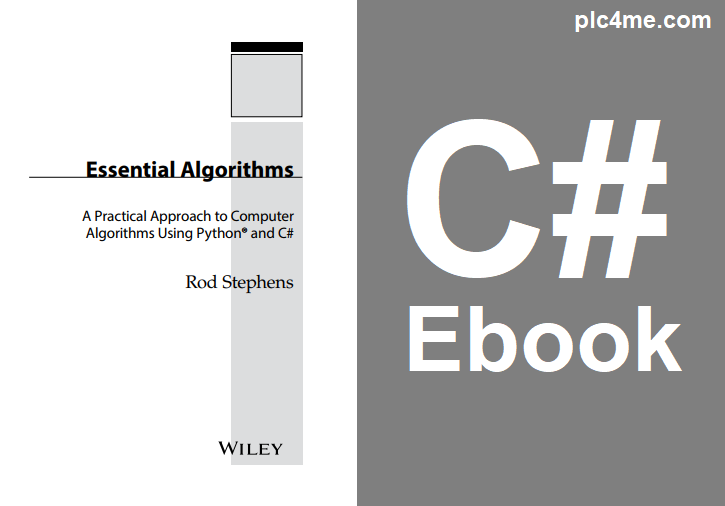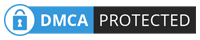C/C++/C# Ebook

# [PDF] Essential Algorithms A Practical Approach to Computer Algorithms Using Python and CWritten by

Algorithms are the recipes that make efficient programming possible. They
explain how to sort records, search for items, calculate numeric values such as prime factors, find the shortest path between two points in a street network, and determine the maximum flow of information possible through a communications network. The difference between using a good algorithm and a bad one can mean the difference between solving a problem in seconds, hours, or never.

Studying algorithms lets you build a useful toolkit of methods for solving
specifc problems. It lets you understand which algorithms are most effective under different circumstances so that you can pick the one best suited for a particular program. An algorithm that provides excellent performance with one set of data may perform terribly with other data, so it is important that you know how to pick the algorithm that is the best match for your scenario.

Even more important, by studying algorithms, you can learn general problemsolving techniques that you can apply to other problems—even if none of the algorithms you already know is a perfect ft for your current situation. These techniques let you look at new problems in different ways so that you can create and analyze your own algorithms to solve your problems and meet unanticipated needs.

#### Content

+ Chapter 1 Algorithm Basics

+ Chapter 2 Numerical Algorithms

+ Chapter 4 Arrays

+ Chapter 5 Stacks and Queues

+ Chapter 6 Sorting

+Chapter 7 Searching

+ Chapter 8 Hash Tables

+ Chapter 9 Recursion

+ Chapter 10 Trees

+ Chapter 11 Balanced Trees

+ Chapter 12 Decision Trees

+ Chapter 13 Basic Network Algorithms

+ Chapter 14 More Network Algorithms

+ Chapter 15 String Algorithms

+ Chapter 16 Cryptography

+ Chapter 17 Complexity Theory

+ Chapter 18 Distributed Algorithms

+ Chapter 19 Interview Puzzles